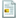## Viewing single post

Started by Legend, Oct 23, 2018, 03:57 PM##### Dec 17, 2018, 11:08 AMLast Edit: Apr 17, 2019, 12:37 AM by xaex Reason: Added new code to list
Wow, the game keeps looking better and better with each screen grab.

I couldn't find any codes for the game anywhere online so I messed around with a bunch of code variations and here's what I've found so far:

 PUZZLE CODE TO ACCESS WHERE CODE CAN BE FOUND • Mole Cube U U D D L L R R O X Scrolls in the Background of Puzzle Selection Screen ? • Slice Cube U U U L L L O O O X Found in 3x3x3 Stats • Baby Infinity Cube D L L R R R O X Found 'coded' on the side of the Mole Cube's orange center • Infinity Cube U D D L L L R R R R O X Found 'coded' on the side of the Mole Cube's orange center • Master Infinity Cube U U D D D L L L L R R R R R O X Found 'coded' on the side of the Mole Cube's orange center • Fragment Cube U U U U D D D D O X Found inside Sol 17 • Corruption Cube U U U D D D U U U D D D O X Found inside Sol 25 • Binary Corruptions U U D D U U D D U U D D O X Found in the RWB Corner of Luna 25 • Magic 3x3x3s A U D L R U D L R O X • Magic 3x3x3s B U D L R U D L R O U D L R O X Found on one of the Mole Cube's eyes • 4x4x4 U R D L U R D L O X • 4x4x4 with Super Edges! U L D R U R D L O X • Bandaged 48 Cube O O O L L L U U U O X • Checkerboard Mixup O O O O O O X • Checkerboard Mixup Plus O O O O O O D O X • Binary Checkerboard Planets O O O O O O D D D O X • Bubble U O U O U O U O X • Chop-O-Matic U U D D L R L R O X • Imposter Cube R L R L D D U U O X • Corner Cube L O R O U O D O X Found 'coded' on one of the Mole Cube's eyes • 1x3x3 O L O O O L O O O X Found via Version 1.06 Update History • 1x3x3 Super O L O O O L O O O R O X Found via Version 1.06 Update History • 2x4x4 Super O O L O O O O L O O O O R O X Found via Version 1.06 Update History • Stubby Pentagon U L D R U L D R O X • Pentultimate L L L L L L L L L O X • Tricky Tower D U D U R R L L O X • 1x2x2 O U U L L O X • Strange 3x3x3 L L U U O O X • Dodecahedron U L D R U L D R O U L D R O X • Mixup Cube Plus 2 O O O O O O D D O X Found BY RandomG • 2x2x2 Squared D D R U U L D D R U U L O X Found BY RandomG

All codes are input at the Puzzle Selection Screen.

I'd also like to note the with the current version of the game the L1 button also = UP, the OPTIONS button = DOWN, the SQUARE button = LEFT, and the TRIANGLE button = RIGHT.

The "CATAMARAN LOCOMOTOR" is found on one of the Mole Cube's eyes. I've tried replacing the C A T N with variations of U D L R and leaving the M as the "middle", but no luck so far. I have some other ways in mind that I'll try out.

I guess the Mole Cube code appears if you stay idle on the Puzzle Selection Screen? I can't seem to get it to show up again after inputting the code and solving the puzzle.

Hope everyone has fun with the new puzzlesI'm having a blast with them (especially the 4x4x4, 4x4x4 with Super Edges!, Bubble and Dodecahedron).

EDIT: Just noticed that I said "1x1x3" in my previous post, I meant "1x3x3"